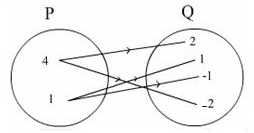Courses

Test: Relations

10 Questions MCQ Test Mathematics For JEE | Test: Relations

Description
Attempt Test: Relations | 10 questions in 10 minutes | Mock test for JEE preparation | Free important questions MCQ to study Mathematics For JEE for JEE Exam | Download free PDF with solutions
QUESTION: 1

Let A = {1, 2, 3, …, 50} and R be the relation “is square of” on A. Then R as a subset of A x A is written as:

Solution:

Since x is the square of y in relation R.
i.e. option D is correct.

QUESTION: 2

Which of the following statement is false?

Solution:

Option D: The number of relations from A to B is 23*2= 26

QUESTION: 3

If n(A) = p and n(B) = q, then how many relations are there from A to B?

Solution:

Number of relation from A to B = 2n(A)×n(B)

n(A) = p, n(B) = q
⇒ Number of relation from A to B  = 2pq

QUESTION: 4

Let R be a relation defined on the set of N natural numbers as R = {(x, y): y is a factor of x, x, y∈ N} then,

Solution:

R = {(x, y): y is a factor of x, x, y∈ N}
As we know that 2 is a factor of 4
So, according to the options (4, 2) ϵ R

QUESTION: 5

If A = {1, 2, 3}, and B = {3, 6} then the number of relations from A to B is

Solution:

The number of relations between sets can be calculated using 2mn where m and n represent the number of members in each set.
So, number of relations from A to B is 26.

QUESTION: 6

Let R be a relation defined as R = {(x, y): y = 2x, x is natural number < 5} then Range of R is given as ,

Solution:

X is a natural number and x < 5, the number is 1, 2, 3, 4
y = 2x

► x(1), y = 2 × 1 = 2
► x(2), y = 2 × 2 = 4
► x(3), y = 2 × 3 = 6
► x(4), y = 2 × 4 = 8

Range = {2, 4, 6, 8}

QUESTION: 7

A relation R defined on the set A = {1, 2, 3, 5} as {(x, y): x + y >10 : x, y ∈ A} is:

Solution:

A = {1, 2, 3, 5}
R = {(x, y): x + y >10: x, y ∈ A}
For any value of x, y ∈ A, the condition does not hold.
Therefore, R = Φ (Empty set)

QUESTION: 8

If A = {1, 2, 3}, B = {4, 5, 6}, which of the following is not a relation from A to B

Solution:

A × B = {(1, 4), (1, 5), (1, 6), (2, 4), (2, 5), (2, 6), (3, 4), (3, 5), (3, 6)}

QUESTION: 9

If n(A) = 2, n(B) = 2. What is the total number of relations from A to B?

Solution:

No. of relations from set A to set B = 2n(A)n(B) =  2(2*2) = 16

QUESTION: 10

The relation shown in the arrow diagram in set builder form isSolution:

Set P is square of the element of set Q.
{(x, y): x is the square of y, x ∈ P, y ∈ Q}Use Code STAYHOME200 and get INR 200 additional OFF Use Coupon Code

Track your progress, build streaks, highlight & save important lessons and more!

Similar ContentRelated tests